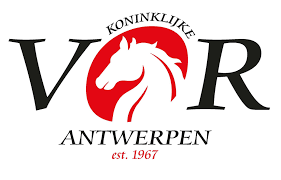Emerald

## 03 6 jarige paarden Barema A - 2 fazen speciaal - 274.2.5

Result list: 59 competitors in this competition. Last updated at 16:54 hrs (05.04.2019)

Rank
Nation
Competitor
CNR
Horse
 Phase 1Fehler Phase 2Fehler TotalFehler Zeit
1
 0 0 = 0.00 31.02
2
 0 0 = 0.00 34.81
3
 0 0 = 0.00 36.55
4
 0 0 = 0.00 37.15
5
 0 0 = 0.00 37.47
6
 0 0 = 0.00 37.71
7
 0 0 = 0.00 38.02
8
 0 0 = 0.00 38.18
9
 0 0 = 0.00 38.23
10
 0 0 = 0.00 38.29
11
 0 0 = 0.00 38.42
12
 0 0 = 0.00 38.61
13
 0 0 = 0.00 38.88
14
 0 0 = 0.00 39.19
15
 0 0 = 0.00 39.27
16
 0 0 = 0.00 39.68
17
GITTE BARTHOLOMEEUSEN,
 0 0 = 0.00 39.7
18
 0 0 = 0.00 40.96
19
 0 0 = 0.00 41.31
20
 0 0 = 0.00 41.53
21
 0 0 = 0.00 41.67
22
 0 0 = 0.00 41.71
23
 0 0 = 0.00 41.86
24
 0 0 = 0.00 42.43
25
 0 0 = 0.00 42.73
26
 0 0 = 0.00 42.84
27
 0 0 = 0.00 42.86
28
 1 0 = 1.00 41.81
29
 0 1 = 1.00 43.79
30
PIETER VAN DEN BERGH,
 0 1 = 1.00 44.83
31
 0 4 = 4.00 35.98
32
 0 4 = 4.00 37.41
33
 4 0 = 4.00 37.87
34
MACKENZIE VAN OETEREN,
 4 0 = 4.00 39.58
35
 0 4 = 4.00 40.15
36
 0 4 = 4.00 41.42
37
 0 4 = 4.00 41.88
38
 4 0 = 4.00 41.92
39
 0 4 = 4.00 41.94
40
 4 0 = 4.00 41.97
41
 0 4 = 4.00 42.27
42
 0 5 = 5.00 43.48
43
 4 1 = 5.00 43.77
44
 4 1 = 5.00 43.84
45
 0 5 = 5.00 44
46
 0 5 = 5.00 44.86
47
 0 5 = 5.00 46.41
48
 4 4 = 8.00 38.74
49
 4 4 = 8.00 40.62
50
 4 4 = 8.00 42.22
51
 8 0 = 8.00 42.56
52
 4 5 = 9.00 43.59
53
 4 7 = 11.00 52.7
54
 8 4 = 12.00 38.06
55
 8 4 = 12.00 39.03
56
 9 4 = 13.00 41.93
57
 4 9 = 13.00 46.41
58
 11 5 = 16.00 46.73
 9 0 eliminated